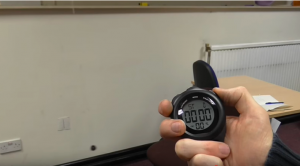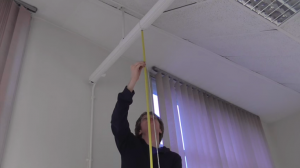# Measuring π with a pendulumFriends of the Aperiodical, nerd-comedy troupe Festival of the Spoken Nerd, are currently on tour around the UK. As part of their show, questionably titled You Can’t Polish a Nerd, Matt Parker attempts to calculate the value of $\pi$ using only a length of string and some meat encased in pastry. He’s previously done this on YouTube, and the idea was inspired by the Aperiodical’s 2015 Pi Approximation Challenge, and in particular my own attempt to approximate $\pi$ with a (more conventional) pendulum.

### The MathsFor our $\pi$ approximate-off, we wanted to derive values for $\pi$ using a suite of methods that mostly didn’t involve measuring the circumference and diameter of a circle. This included evaluating as many terms of some infinite series as the length of our room booking permitted, and a recreation of the famous Buffon’s Needle experiment. But surely the most satisfying method is to just swing a heavy ball on a bit of string.As many people learn in high school, the formula governing how long a pendulum takes to do one complete swing is $T = 2\pi\sqrt{\frac l g}$ where $l$ is the length of the pendulum and $g$ is the acceleration due to gravity (about $9.8ms^{-2}$). Slightly counterintuitively, the swing time doesn’t depend on the weight of the bob (or if you prefer, the mass of the weight), nor on how far back you swing it from. So just from timing a swing and measuring the string, you can solve the equation to get a numerical value for $\pi$.But all that calculation sounds pretty boring. We can choose the length of the string, so why not choose it so that it cancels the $l$ and the 2, to give $T=\pi$? Conveniently that requires a length of $g/4 \approx 2.45$ metres (if you’ll excuse my abuse of dimensionality), which is just about doable in a tallish room or a stairwell or a theatre stage. When we tried this timing ten swings to reduce the impact of imperfect human reaction time, we ended up with a value of 3.133. (I speculate that the overwhelming source of inaccuracy is the difficulty in measuring the length of the string from the precise point of pivot at the top to the precise centre of mass of the bob/weight/pie.) On his video, Matt, using a fancy slow-mo camera, got a value of 3.128 seconds per swing. The Aperiodical has yet to take its annual pilgrimage to the Festival, so it remains to be seen how successfully the feat is accomplished live.

### But it’s just more circles, right?

You might think that using a pendulum in an attempt to find $\pi$ without using circles is cheating a bit: surely a pendulum’s swing time depends on $\pi$ because it moves along the arc of a circle? Well, no. In fact the opposite is true. I lied a bit when I said the time doesn’t depend on how far back you pull the pendulum. The formula above is only an approximation for small initial angles, when a pendulum approximates simple harmonic motion. The full formula is this:So in fact the more of a circle you make your pendulum trace out, the worse your approximation will be. Circles kill pi.

If you’d like to see Matt attempt this live on stage, the Spoken Nerd show is still on tour until the end of November, and as well as swinging food, it includes songs, live experiments, comedy and many nerdy references.

Calculating π with a pendulum, by Matt Parker on YouTube
Aperiodical’s π approximation challenge, on YouTube
Festival of the Spoken Nerd tour

## About the author

•#### Paul Taylor

Maths graduate, inveterate crossword and general puzzle doer, uses brackets excessively in writing (sorry)